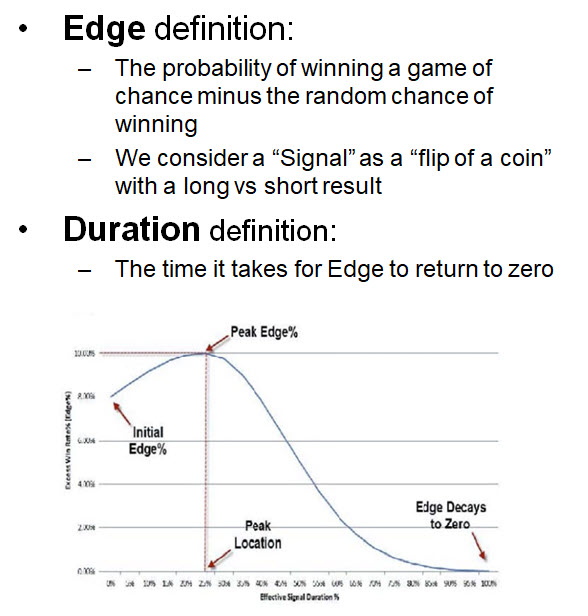## Predictor Validation

Parallax has been producing scientifically derived forecasting tools since 1990, so it is an integral part of our business to validate these predictors using reliable statistical methods. With each predictor, we need to answer the following set of questions:

• What price behavior is being predicted?
• What is the effective duration of the predictor?
• How statistically significant is the predictor at each time step forward?
• Is the predictor effective on all time scales?
• Is the predictor effective on all financial series?

Evaluating a predictor is a different process from evaluating full trading systems. We use some math most often found in casinos. In a casino, it’s said that the house has the edge, meaning that the probability of winning one of their games consistently over time is less than 50%.

We define the predictive edge as the win rate of our predictor minus the random background edge. This is a scale-independent measure. Obviously, to be useful, the edge must differ significantly from the background and have a long enough duration to be profitable. To carry out this analysis, it is necessary to measure the price action during the time period immediately following each signal. We call this the “post-predictor” or “outrun” period. To get a full picture, we process thousands of each signal type on multiple time scales.We use a simple model to find the predictive edge and duration for each of our forecasting tools

It’s most important to know exactly what is being predicted by the tool being evaluated. For instance, an ExtremeHurst Compression predicts that the market in question should cease mean-reverting sideways and enter a new persistent trend either up or down. This means that we need to measure a scale-independent absolute value of trend change. We built the TMI indicator for this purpose. The graph below shows that thousands of compression signals produce statistically significant trend changes compared to the background. A Chi-squared statistical test showed this was significant to p < 1.27 x 10^-177